KUULCHAT
MATHEMATICS MOCK

#### OBJECTIVE TEST

1.

If the bearing of A from B is 240o, find the bearing of B from A

A.

040o

B.

060o

C.

120o

D.

300o

2.

A bag contains 5 red and 7 black balls of the same size. What is the probability of picking a black ball?

A.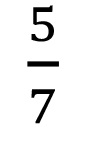B.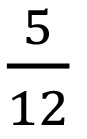C.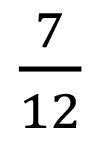D.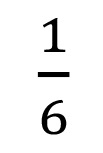3.

Solve: 5x - (7x - 3) ≤ 9.

A.

x ≥ -3

B.

x ≤ -3

C.

x ≥ -6

D.

x ≤ 3

4.

Solve:A.

4

B.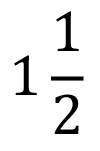C.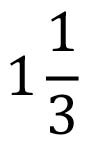D.

0

5.

A man travelled a distance of 8 km in an hour. How long will it take him to cover a distance of 12 km, travelling at the same speed?

A.

1⅓ hrs

B.

1½ hrs

C.

1¾ hrs

D.

2 hrs

6.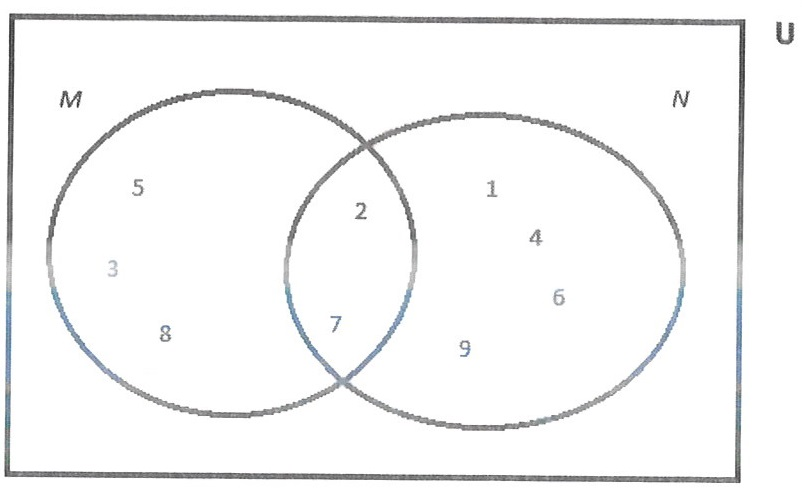In the Venn diagram M and N are the subsets of the universal set U.

Use this information to answer the question below.

How many members are in the set N?

A.

2

B.

3

C.

4

D.

6

7.

Factorize: 3ax + 6a - x - 2

A.

(3a+1)(x+2)

B.

(3a+1)(x-2)

C.

3a(x-2)

D.

(3a-1)(x+2)

8.

Divide 0.5445 by 0.09.

A.

5.05

B.

6.05

C.

6.50

D.

60.50

9.

Make h the subject of the relation V = πr2h.

A.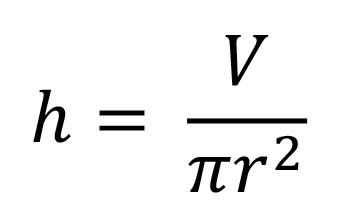B.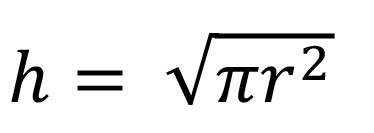C.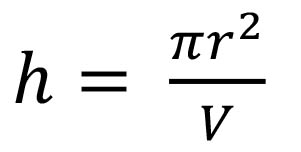D.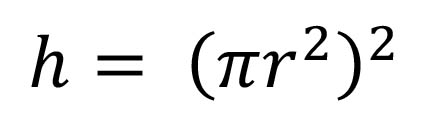10.

Express 134.78 correct to the nearest tenth

A.

130.0

B.

134.7

C.

134.8

D.

135.0

11.

Which of the following is arranged in ascending order?

A.

-25, -64, 4, 17

B.

-64, -25, 4, 17

C.

-64, -25, 17, 4

D.

17, 4, -25, -64

12.

A trader sold a radio set for GH₵ 72.00 making a profit of 8%. Find, correct to the nearest Ghana cedi, the cost of the radio set.

A.

GH₵ 66.00

B.

GH₵ 67.00

C.

GH₵ 77.00

D.

GH₵ 78.00

13.

Subtract (7x-3) from (5-3x).

A.

10x-8

B.

4x-8

C.

8-10x

D.

2-10x

14.

The cost of 12 note books is GH₵ 54.84. Find the cost of one note book.

A.

GH₵ 5.57

B.

GH₵ 4.67

C.

GH₵ 4.57

D.

GH₵ 3.57

15.

Ama bought a pair of sandals for GH₵ 20.00 and solid it at GH₵ 24.00. Find the percentage profit.

A.

4 %

B.

17 %

C.

20 %

D.

44 %

16.

Evaluate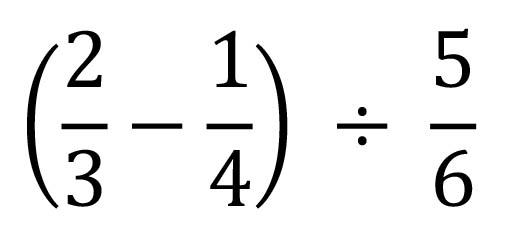A.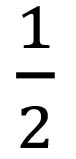B.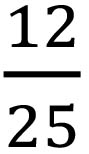C.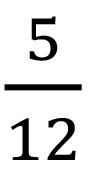D.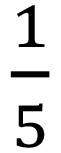17.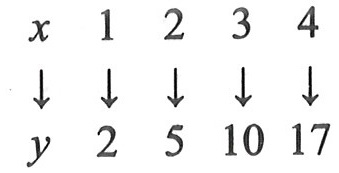What is the rule for the mapping?

A.

x→ 3x2-1

B.

x→ 5x2-3

C.

xx2+1

D.

x→ 4x-2

18.

The simple interest on GH₵ 450.00 for 4 years is GH₵ 45.00, find the rate of interest

A.

2.5 %

B.

10 %

C.

25 %

D.

6.5 %

19.

Correct 0.024561 to three significant figures.

A.

0.03

B.

0.025

C.

0.0245

D.

0.0246

20.

Arrange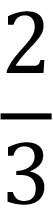,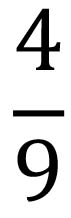and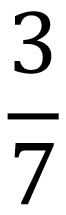in ascending order.

A.,,B.,,C.,,D.,,21.

Which property of arithmetic is used in a(x+y) = ax + ay.

A.

Associative

B.

Commutative

C.

Distributive

D.

Initiative

22.

Two sides of a rectangle are 10 cm and 6 cm. Calculate the area of a square with the same perimeter as that of the rectangle.

A.

16 cm2

B.

30 cm2

C.

60 cm2

D.

64 cm2

23.

Which of the following is an infinite set?

A.

{1, 2, ..., 5, 6, 7}

B.

{4, 6, 8, 10, 12}

C.

{2, 3, 5, 7, 11, ...}

D.

{3, 6, ..., 18, 21, ...33, 36}

24.

Find the rule of the mapping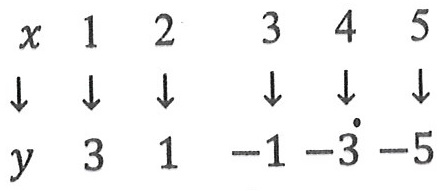A.

y = 2x + 2

B.

y = -2x + 2

C.

y = 4x

D.

y = -2x + 5

25.

Find the gradient of the straight line which passes through the points (-3,4) and (3,-2).

A.

2

B.

1

C.

-2

D.

-1

26.

Find the image of the point (-2,3) under a reflection in the y-axis.

A.

(2,-3)

B.

(-3,2)

C.

(2,3)

D.

(3,2)

27.

Find the median of the following numbers: 46, 68, 34, 37, 76 and 81.

A.

35.5

B.

57

C.

67

D.

68

28.

Solve the equation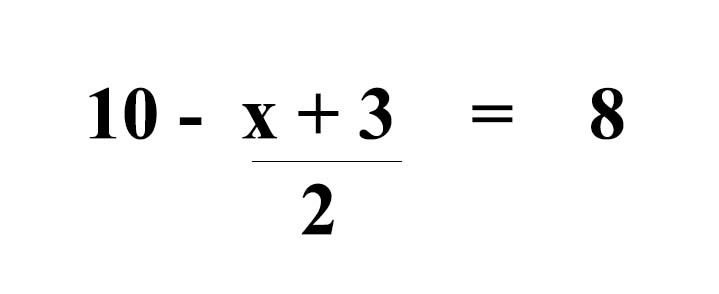A.

-9

B.

-3

C.

1

D.

15

29.

Find the least number that can be added to 207 to make the sum divisible by 17.

A.

3

B.

13

C.

14

D.

30

30.

Evaluate: (0.07 x 0.02) ÷ 14.

A.

0.01

B.

0.001

C.

0.0001

D.

0.00001

31.

A car covered a distance of 150 km at a speed of 18 km/h. Find the time taken.

A.

7 hours 33 minutes

B.

7 hours 53 minutes

C.

8 hours 13 minutes

D.

8 hours 20 minutes

32.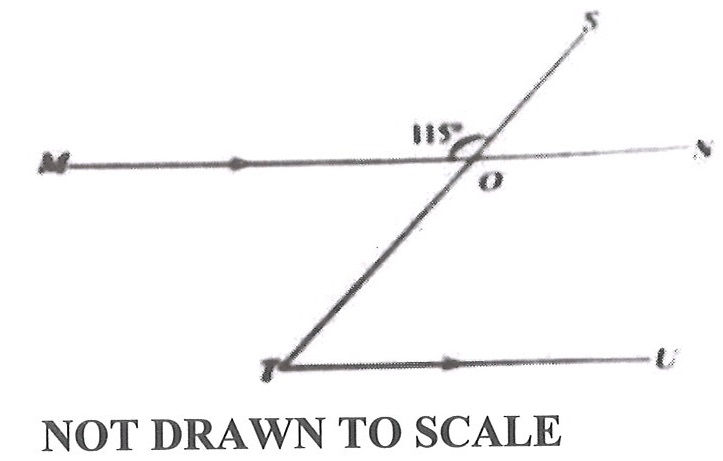In the diagram, line MN is parallel to line TU, line TS cuts line MN at O and ∠MOS = 115o. Find ∠OTU.

A.

65o

B.

55o

C.

45o

D.

25o

33.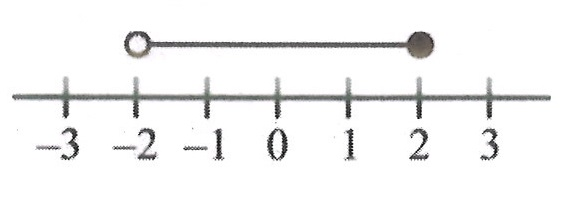Which of the following inequalities is represented on the number line?

A.

-2>y>2

B.

-2≤ y < 2

C.

-2 ≥ y > 2

D.

-2 < y ≤ 2

34.

A football match starts at 2.20 p.m. and lasts for 1 hour 50 minutes. At what time will the game end?

A.

3.10 p.m.

B.

4.10 p.m.

C.

5.10 p.m.

D.

6.10 p.m.

35.

On a map, 1⁄3 cm represents 5km. If two towns A and B are 18 cm apart on the map, what is the actual distance between them?

A.

27 km

B.

30 km

C.

240 km

D.

270 km

36.

If P = {x:x is an even number greater than two and less or equal to twelve}, list the members of P

A.

{2, 4, 2, 8, 10, 12}

B.

{3,4,6,8,10,12}

C.

{2, 4, 6, 8, 10}

D.

{4, 6, 8, 10, 12}

37.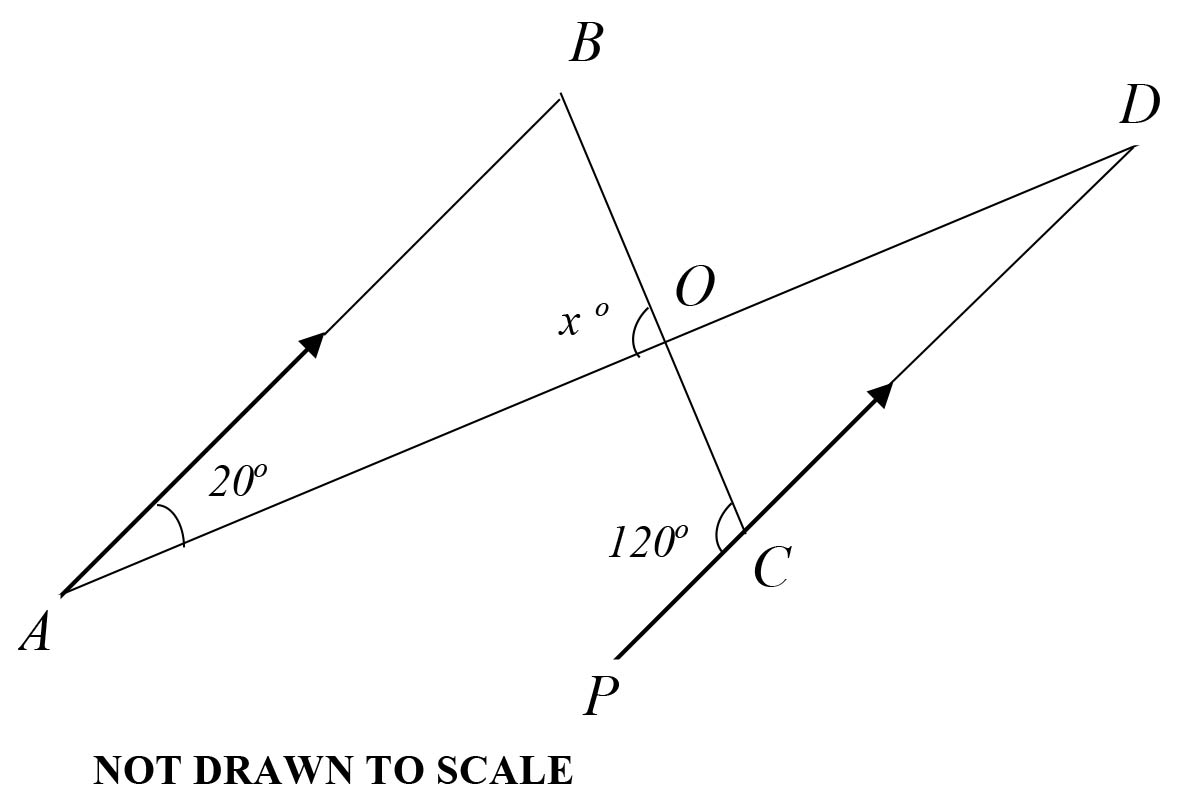In the diagram, line AB is parallel to line PD. Find the value of x.

A.

20o

B.

80o

C.

100o

D.

120o

38.

Which of the following numbers is the largest?

A.

-70

B.

-50

C.

-3

D.

-2

39.

Given that x = 4, y = 7, evaluate 2xy + 3(x+y)

A.

79

B.

89

C.

99

D.

109

40.

Express 0.725 as a fraction in its lowest term.

A.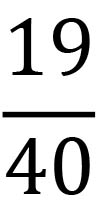B.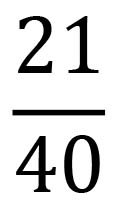C.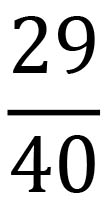D.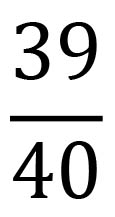#### THEORY QUESTIONS

1.

The marks obtained by students in a class test were

 4 8 7 6 7 2 1 7 4 7 3 7 6 4 3 7 5 2 7 2 5 4 8 3 2

a

Construct a frequency distribution table for the data.

b

Find the:

i)

mode of the distribution

ii)

median mark of the test;

iii)

mean mark.

2.

a

Simplify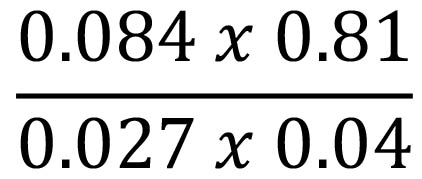, leaving the answer in standard form.

b

i)

Make r the subject of the relationii)

From (b)(i), find the value of r when y = 3 and x = 10.

c

Juliet bought 1,756 kg of frozen chicken, 675 g of vegetables and 95 g of corn oil from a Shopping Mall. What is the total weight of the items she bought in kilogram?

3.

a

An English textbook costs GH₵ 25.00. The author of the book agreed to take 20% of the cost of each book sold. If 1,702 copies were sold, calculate the author's share.

b

Simplify: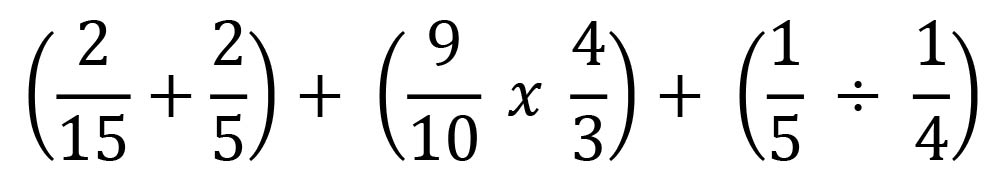c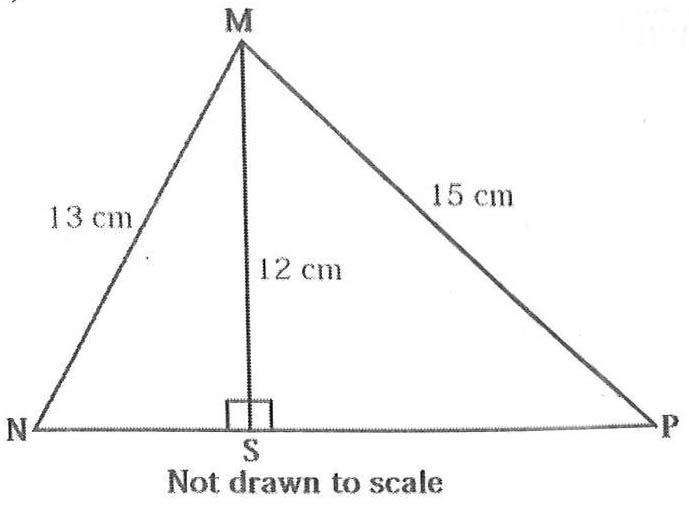In the diagram, MN = 13 cm, MP = 15 cm, MS = 12 cm and MS is perpendicular to NP.

Calculate length NP.

4.

a

 x 1 2 3 4 5 ↓ ↓ ↓ ↓ ↓ ↓ y 0 3 6 9 12

The mapping shows the relationship between x and y.

i)

using a scale of 2 cm to 1 unit on the x-axis and 2 cm to 2 units on the y-axis, draw two perpendicular axes 0x and 0y on a graph sheet for 1 ≤ x ≤ 5 and 0 ≤ x ≤ 14;

ii)

plot the point for each ordered pair, (x, y).

iii)

join the points with a straight line;

iv)

using the graph, find the gradient of the line in (a)(iii);

v)

use the graph to find the equation of the line in (a)(iii).

b

Simplify: 32 x 8 x 4 x 2, leaving the answer in the form 2n

5.

a

In an examination 60 candidates passed Integrated Science or Mathematics. If 15 passed both subjects and 9 more passed Mathematics than Integrated Science, find the:
i) number of candidates who passed in each subject;
ii) probability that a candidate passed exactly one subject.

b

Factorize: xy + 6x + 3y + 18

6.

a)

Simplify: 5(6 - ab) + 2(-7 + 3ab)

b)

The equation of a straight line is given by 3x - 2y - 6 = 0. Find the: